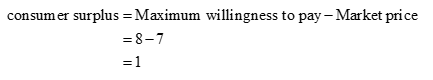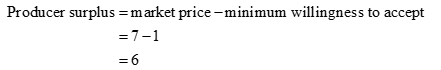# Suppose we have a product with the following conditions:The consumer's maximum willingness to pay is 8A firm's minimum willingness to accept is 1The market price is 7a. What is the consumer surplus?CS =b. What is the producer surplus?PS =c. What is the total surplusTS =

Question
1 views

Suppose we have a product with the following conditions:

The consumer's maximum willingness to pay is 8

A firm's minimum willingness to accept is 1

The market price is 7

a. What is the consumer surplus?

CS =

b. What is the producer surplus?

PS =

c. What is the total surplus

TS =

check_circle

Step 1

a) Consumer surplus is calculated as below:Step 2

b) Producer Surplus is calculated as :...

### Want to see the full answer?

See Solution

#### Want to see this answer and more?

Solutions are written by subject experts who are available 24/7. Questions are typically answered within 1 hour.*

See Solution
*Response times may vary by subject and question.
Tagged in